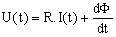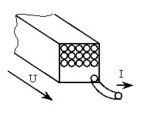# Stranded conductor component

## Introduction

From the physical point of view, a stranded conductor consists of one or more thin wire elementary conductors. The diameter of the wire is lower than the skin depth, so that the current density is practically constant over all the conductor cross-section.

## Stranded conductor: representation

A stranded conductor is represented:

• in the finite elements domain by a region of stranded conductor type (or by a non-meshed coil in 3D)
• in the electrical circuit by a component of stranded conductor type

## Stranded conductor: relation U, I, Φ

The basic relation linking the current I, the voltage U and the flux Φ is given below.

The current flowing through a stranded conductor component is the current I flowing through each strand (turn) of the correspondent coil.

The voltage at the terminals of a stranded conductor component is the voltage U at the terminals of the modeled assembly of the coil strands.where:

• R is the resistance of the coil
• Φ is the flux embraced by the assembly of the coil strands## Resistance

The resistance R of a stranded component is equal to the resistance of the coil strands assembly:

R = n⋅Rstrand = n⋅(ρ⋅l / Sstrand)

The cross section of a strand is equal to:

Sstrand = F⋅Sregion /n

with :

• ρ: material resistivity
• l: length of a strand, viz. the depth of the modeled device in 2D
• F: space factor (0 < F < 1) (area of the surface filled by the strands / area of the modeled region surface)
• n: number of strands